# Solid Cylinder, Steady 2D, R0JZKL

Consider two-dimensional heat conduction in the finite cylinder. The temperature is given by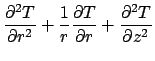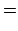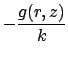(12)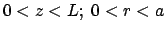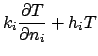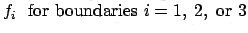The temperature may be stated in the form of integrals with the method of Green's functions. If the Green's function,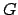, is known, the temperature is given by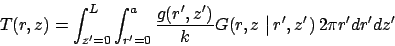(for volume energy generation)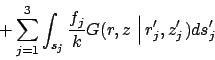(for boundary conditions of type 2 and 3)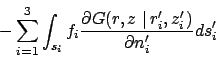(13)

(for boundary conditions of type 1 only).

The same Green's function appears in each integral but is evaluated at locations appropriate for each integral. Here position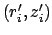is located on surface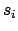. Surface differential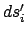is associated with appropriate surfaces of the cylinder: on surface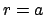,; and, at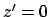or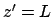,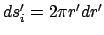.

GF, 2D Cylinder

The steady Green's function represents the response at point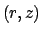caused by a point source of heat located at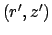. The GF for the finite cylinder satisfies the following equations: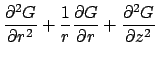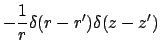(14)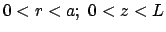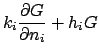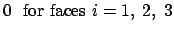Note that the boundary conditions are homogeneous and of the same type as the temperature problem.

The Green's function for the cylinder with axisymmetric heat conduction is given by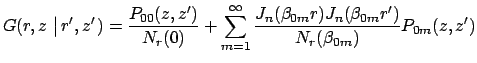(15)

where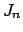are Bessel functions of order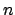. The norms, eigenconditions, and kernel functions are identical to those used for the three-dimensional GF. The above single-sum GF may be derived either by a direct solution of the defining equation for, or, by integrating the 3D GF over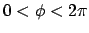. A physical interpretation of this approach, called the method of descent, is to distribute 3D point sources to form a ring-shaped source appropriate for axisymmetric 2D heating. A double-sum form of the GF may also be found from the transient GF by the limit method; see Beck et al. (1992, p. 249) for a discussion of case R01Z11.